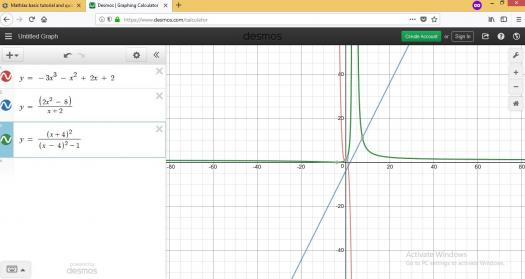# Basic Calculus

4 posts / 0 new
Help_me_learn_Maths
Basic Calculus

Help me find the limits of these:

https://imgur.com/a/yobgF

Help me identify all asymptotes and the end behavior of the graph:

https://imgur.com/a/JxJF2

Tags:
fitzmerl duron$\mathbf{For \space the \space first \space part}$

Answers for numbers $1$ to $7$.

$1.$ I don't know. Hindi ko mabasa yung equation eh...hehe

$2.$ Limit is $1$.

$3.$ No limit. Limit is very big. Infinite, to be exact.

$4.$ Limit is $2$.

$5.$ No limit. Limit is very big. Infinite, to be exact.

$6.$ Limit is $\frac{3}{2}$

$7.$ Limit is $0.$

I will show the solution for numbers $6$ and $7$ and leave the remaining numbers as an exercise for you...

To get the limit of item $6$, which is $\lim_{x\to \infty}\frac{3x^4 - 2x^2 + 3x + 1}{2x^4 - 2x^2 + x - 3}$, the evaluation of limits as $x \rightarrow \pm \infty$ is most easily accomplished, if possible, by expressing the function in terms of $\frac{1}{x}$ and using the fact that $\frac{1}{x} \rightarrow 0$ as $x$ approaches infinity.

With that in mind, we do this now...

$$\lim_{x\to \infty} \frac{3x^4 - 2x^2 + 3x + 1}{2x^4 - 2x^2 + x - 3}$$ $$\lim_{x\to \infty} \frac{\frac{3x^4}{x^4} - \frac{2x^2}{x^4} + \frac{3x}{x^4} + \frac{1}{x^4}}{\frac{2x^4}{x^4} - \frac{2x^2}{x^4} + \frac{x}{x^4} - \frac{3}{x^4}}$$ $$\lim_{x\to \infty} \frac{3 - \frac{2}{x^2} + \frac{3}{x^3} + \frac{1}{x^4}}{2 - \frac{2}{x^2} + \frac{1}{x^3} - \frac{3}{x^4}}$$ $$= \frac{3 - 0 + 0 + 0}{2 - 0 + 0 - 0}$$ $$\color{green}{\frac{3}{2}}$$

The limit of $\lim_{x\to \infty} \frac{3x^4 - 2x^2 + 3x + 1}{2x^4 - 2x^2 + x - 3}$ is $\frac{3}{2}$.

To get the limit of item $7$, which is $\lim_{x\to \infty} \frac{2x^2 - x + 3}{x^5 - 2x^3 + 2x - 3}$, we'll try subtituting $\infty$ into a value very big enough to get the limit of item $7$. We choose $9999999999$ instead of $\infty$ so that we can input it into the calculator.

Armed with these, we do this now...

$$\lim_{x\to \infty} \frac{2x^2 - x + 3}{x^5 - 2x^3 + 2x - 3}$$

becomes...

$$\lim_{x\to 9999999999} \frac{2x^2 - x + 3}{x^5 - 2x^3 + 2x - 3}$$

Then...

$$\lim_{x\to 9999999999} \frac{2x^2 - x + 3}{x^5 - 2x^3 + 2x - 3}$$ $$= \frac{2(9999999999)^2 - (9999999999) + 3}{(9999999999)^5 - 2(9999999999)^3 + 2(9999999999) - 3}$$ $$\approx 0$$

Therefore, the limit of $$\lim_{x\to \infty} \frac{2x^2 - x + 3}{x^5 - 2x^3 + 2x - 3}$$ is $\color{green}{0}$

$\mathbf{For \space the \space second \space part}$

I will just answer items $9$ and $10$ and leave number $8$ as your exercise...Looking at the two equations $\color{red}{y = -3x^3 - x^2 + 2x + 2}$ and $\color{blue}{y = \frac{2x^2 - 8}{x + 2}}$ and their graphs, it become clear. Both equations $\mathbf{don't \space have \space asymptotes}$ because both equations are continuous functions. What do you conclude if you're looking at the graph of $\color{green}{y = \frac{(x+4)^2}{(x-4)^2 -1}}$?

Altenate solutions are highly encourage....:-)

Help_me_learn_Maths

The problem in number 1 is same as the number 2 but below the lim is x->8^+
But, my answer for is limit = 3 not sure if it is correct tho.

x = =5, x = -3 is this correct? How about the end behavior what is that?

fitzmerl duronIf $x = 5$, that would make the denominator zero. If $x = -3$, it would make the denominator valued at 48. Therefore, there is an asymptote at the line $x = 5$ in the equation number 8. The equation of the asymptote is $x = 5$. Describing the end-behavior of the graph of equation $8$, there is a discontinuity at $x = 5$. The left and right portions of $x = 5$ are smooth...

• Mathematics inside the configured delimiters is rendered by MathJax. The default math delimiters are $$...$$ and $...$ for displayed mathematics, and $...$ and $...$ for in-line mathematics.